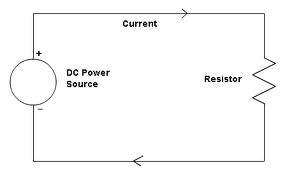## Maximum Power Transfer in Case of DC Network Assignment Help

Assignment Help: >> Maximum Power Transfer Theorem - Maximum Power Transfer in Case of DC Network

Maximum Power Transfer in Case of DC Network:

Figure, illustrates the DC network for maximum power transfer. The load current is given by

iL  = VTH / RTH + RLPower delivered to load

PL = (iL )2 × R = ( (VTH )2 /(R TH + R  )2 )× RL

Condition for maximum power

dPL/ dRL       = 0

⇒         RL = RTH

So load resistance must be equal to the internal resistance of circuit for receiving the maximum power.

The maximum power from equation

Pmax  =( VTh ) 2/(RL+ RL )× RL   = VTH /4 RL

P max     =          VTH   /4 RTH

Maximum power transfer theorem is useful in communication network and electronic circuits where demand of maximum power is important instead of the efficiency. The total efficiency of a network supplying maximum power to any branch is only 50%. So use of maximum power transfer theorem is restricted in electrical and distribution networks where aim is high efficiency not the maximum power.

As earlier stated, before applying the maximum power transfer theorem, we suppose that circuit must be in Thevenin's equivalent form. So, here we describe the Thevenin theorem and then draw the Thevenin's equivalent circuit.

Thevenin theorem states that any linear bilateral network across its load terminal may be replaced by simple network having one voltage source (VTH) and one series impedance (ZTH).#### Assured A++ Grade

Get guaranteed satisfaction & time on delivery in every assignment order you paid with us! We ensure premium quality solution document along with free turntin report!

All rights reserved! Copyrights ©2019-2020 ExpertsMind IT Educational Pvt Ltd